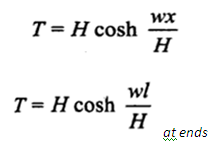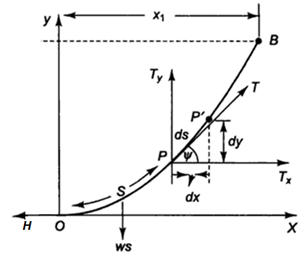# Tension in Transmission line Calculator

This Calctown Calculator calculates the tension in a transmission line.

N
kg/m
m
m

#### Result

N
Nwhere

T = tension at point P

x = horizontal distance of point P from O

l = half span of towers

H = tension at O

w = weight per unit length